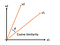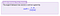# Building a Recommendation Algorithm in Ruby on Rails (Part 2)Cosine Similarity is using the cosine of the angle between two vectors to measure how close they are

## Creating Custom Attribute Values for Each Game

`require 'rest-client'require_relative 'games_array_demo.rb'games_array = get_games`
`games_array.each do |game|Game.create(name: game[:name],image: game[:image],price: game[:price],max_players: game[:max_players],min_players: game[:min_players],play_time: game[:play_time],description: game[:description],genre: game[:genre],rating: game[:rating],rank: game[:rank],board_score: game[:board_score],players_score: game[:players_score],card_score: rand(10),party_score: rand(10))end`

# Actually Building The Algorithm

`def similarity_score_game(game)# create a vector for the game method is being called on# attributes needed are board_score, card_score, party_score, and players_scorevector_a = Vector[self.board_score, self.card_score, self.party_score, self.players_score]#next, create a vector for the game being passed in to the method#we will then compare the two vectors to see how similar the games arevector_b = Vector[game.board_score, game.card_score, game.party_score, game.players_score]#now that we have 2 vectors we will find the similarity by putting the dot product#in our numerator, and the product of each vectors magnitude in our denominatornumerator = vector_a.inner_product(vector_b)denominator = vector_a.r * vector_b.r#this will give the cosine similarityscore = numerator/denominator*100return score.to_i#since cosine is a decimal between 0 and 1, multiplying by 100 and then rounding to the nearest integer gives a nice scoring system from 0 to 100end`The method above is just this formula
`> first = Game.first> second = Game.second> first.similarity_score_game(second)=> 97`

# Recommending Games based on a User’s Profile

## Building a User Profile

`User.create(uname: ‘Sean’,board_score: 5,card_score: 6,party_score: 3,players_score: 3)`
`def create_user_profile#Create vector that represents user profile@total_reviews = 2@user_profile = Vector[self.board_score, self.card_score, self.party_score, self.players_score]end`
`def update_user_profile(new_rating_vector)#mean of user profile and weighted new item review# Vold = current user profile#Vnew = new user profile after rating is factored in#Vitem = vector representation of item being review#If the review is favorable, use it to calculate new mean#If review was negative, use the inverse vector to calculate new mean#ex - If a user profile consists of 5 reviews, the effect of a 6th would#looks like this:#(5 x Vold + Vitem)/6#formula: Vnew = ((n x Vold) + Vitem )/ n + 1new_vector_board = (@total_reviews * @user_profile + new_rating_vector)/(@total_reviews + 1)new_vector_card = (@total_reviews * @user_profile + new_rating_vector)/(@total_reviews + 1)new_vector_party = (@total_reviews * @user_profile + new_rating_vector)/(@total_reviews + 1)new_vector_players = (@total_reviews * @user_profile + new_rating_vector)/(@total_reviews + 1)@user_profile = Vector[new_vector_board, new_vector_card, new_vector_party, new_vector_players]@total_reviews += 1return @user_profileend`
`def similarity_score(game)# create a vector for the user the method is being called on# attributes needed are board_score, card_score, party_score and players_scoreuser_vector = @user_profile#next, create a vector for the game being passed in to the method#we will then compare the two vectors to see how similar the games aregame_vector = Vector[game.board_score, game.card_score, game.party_score, game.players_score ]#now that we have 2 vectors we will find the similarity by putting the dot product in our numerator, and the product of each vectors magnitude in our denominatornumerator = user_vector.inner_product(game_vector)denominator = user_vector.r * game_vector.r#this will give the cosine similarityscore = numerator/denominator*100return score.to_iend`
`def game_recsgame_rec_array = []self.create_user_profileGame.all.each do |game|score = game.similarity_score_user(self)game_rec_array.push({game: game, similarity: score})endranked = game_rec_array.sort_by{|rec| -rec[:similarity]}return rankedend`

NYC based SWE